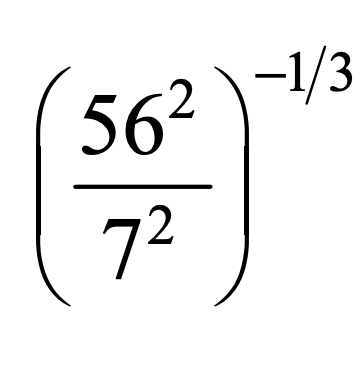Properties of Exponents
Rational Exponents
Solving Rational Equations
Properties of Rational Exponents
100

n^4/n^3

n

100

36^(-1/2)

1/6

100

4x^3=32

x=2

100

Trivia Break!

What animal is known to spend 90% of its day sleeping?

koala

100

(root 3(250))/root3(2)

5

200

x^5/(x^6•x^2)

1/(x^3)

200

Trivia Break!

Of the 7 continents, which contains the most countries?

Africa - there are 54 countries
200

5x^3=1080

x=6

200

Simplify the powers:

(3)/(3^(1/4))

3^(3/4)

200

root3(65y^6

= root3(4^3(y^2)^3 = 4y^2

300

(3s^3)^2/(ts^5)

(9s)/t

300

16^(3/2

= (16^(1/2))^3 =(sqrt16)^3 = 4^3= 64

300

Trivia Break!

In the Little Mermaid, what is the name of the singing  crustacean?

Sebastian

300

Simplify the powers:

((42^(1/3))/(6^(1/3)))^2

7^(2/3)

300

root4(256a^6b^11c^4

2ab^2croot4(a^2b^3)

400

((4w^3)/(2z^2))^3

(8w^9)/(z^6)

400

Rewrite in proper radical form, then solve:

1^(7/8)

1

400

(x-5)^4=256

x=1 and 9

400

Fully Simplify:

(4^5•3^5)^(-1/5)

1/12

400

Trivia Break!

What is the highest grossing movie of all time?

Avatar

500

Trivia Break!

What is the highest score ever recorded by one team in a Superbowl game?

55 points (the 49ers)

500

32^(-3/5)

= ((1)/(root5(32)^3))=1/8

500

2x^4=1250

x=-5 and 5

500500

(14xy^(1/3))/(2x^(3/4)z^(-6))

7x^(1/4)y^(1/3)z^6

Click to zoom